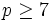# Elementary abelian-to-normal replacement fails for half of prime plus nine for prime greater than five

Suppose$p$ is a prime greater than$5$ (in other words,$p \ge 7$). Suppose$k \ge (p + 9)/2$. Then, there exists a finite$p$-group$P$ that contains an elementary abelian subgroup of order$p^k$ but no elementary abelian normal subgroup of order$p^k$.